xSurface layerEncyclopedia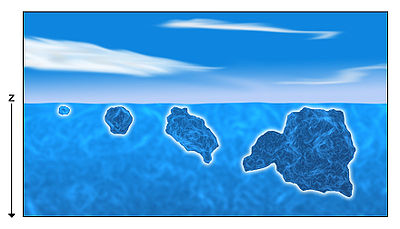The surface layer is the layer of a turbulent fluid most affected by interaction with a solid surface or the surface separating a gas and a liquid where the characteristics of the turbulence
Turbulence
In fluid dynamics, turbulence or turbulent flow is a flow regime characterized by chaotic and stochastic property changes. This includes low momentum diffusion, high momentum convection, and rapid variation of pressure and velocity in space and time...

depend on distance from the interface. Surface layers are characterized by large normal gradient
In vector calculus, the gradient of a scalar field is a vector field that points in the direction of the greatest rate of increase of the scalar field, and whose magnitude is the greatest rate of change....

s of tangential velocity
Velocity
In physics, velocity is speed in a given direction. Speed describes only how fast an object is moving, whereas velocity gives both the speed and direction of the object's motion. To have a constant velocity, an object must have a constant speed and motion in a constant direction. Constant ...

and large concentration gradients of any substances (temperature
Temperature
Temperature is a physical property of matter that quantitatively expresses the common notions of hot and cold. Objects of low temperature are cold, while various degrees of higher temperatures are referred to as warm or hot...

, moisture
Moisture
Humidity is the amount of moisture the air can hold before it rains. Moisture refers to the presence of a liquid, especially water, often in trace amounts...

, sediments et cetera) transport
Transport
Transport or transportation is the movement of people, cattle, animals and goods from one location to another. Modes of transport include air, rail, road, water, cable, pipeline, and space. The field can be divided into infrastructure, vehicles, and operations...

ed to or from the interface.

The term boundary layer is used in meteorology
Meteorology
Meteorology is the interdisciplinary scientific study of the atmosphere. Studies in the field stretch back millennia, though significant progress in meteorology did not occur until the 18th century. The 19th century saw breakthroughs occur after observing networks developed across several countries...

and in physical oceanography
Oceanography
Oceanography , also called oceanology or marine science, is the branch of Earth science that studies the ocean...

. The atmospheric surface layer is the lowest part of the atmospheric boundary layer (typically the bottom 10% where the log wind profile
Log wind profile
The Log wind profile is a semi-empirical relationship used to describe the vertical distribution of horizontal wind speeds above the ground within the atmospheric surface layer...

is valid). The ocean has two surface layers: the benthic, found immediately above the sea floor and the marine
Marine (ocean)
Marine is an umbrella term. As an adjective it is usually applicable to things relating to the sea or ocean, such as marine biology, marine ecology and marine geology...

surface layer, at the air-sea interface
Interface (chemistry)
An interface is a surface forming a common boundary among two different phases, such as an insoluble solid and a liquid, two immiscible liquids or a liquid and an insoluble gas. The importance of the interface depends on which type of system is being treated: the bigger the quotient area/volume,...

.

## Mathematical Formulation

A simple model
Mathematical model
A mathematical model is a description of a system using mathematical concepts and language. The process of developing a mathematical model is termed mathematical modeling. Mathematical models are used not only in the natural sciences and engineering disciplines A mathematical model is a...

of the surface layer can be derived by first examining the turbulent momentum flux through a surface.
Using Reynolds Decomposition to express the horizontal flow in thedirection as the sum of a slowly varying component,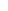, and a turbulent component,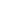,: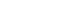and the vertical flow,, in an analogous fashion: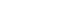we can express the flux of turbulent momentum through a surface,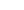as the time averaged magnitude of vertical turbulent transport of horizontal turbulent momentum,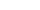: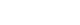.

If the flow is homogeneous within the region, we can set the product of the vertical gradient of the mean horizontal flow and the eddy viscosity coefficient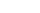equal to: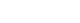,

whereis defined in terms of Prandtl's mixing length hypothesis: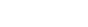where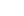is the mixing length.

We can then expressas: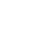.

## Assumptions about the mixing length

From the figure above, we can see that the size of a turbulent eddy near the surface is constrained by its proximity to the surface; turbulent eddies centered near the surface cannot be as large as those centered further from the surface. From this consideration, it is reasonable to assume that the mixing length
Mixing length theory
In fluid dynamics, mixing length model is a method attempting to describe momentum transfer by turbulence Reynolds stresses within a newtonian fluid boundary layer by means of an eddy viscosity. The model was developed by Ludwig Prandtl in the early 20th century...

,is proportional to the eddy's depth in the surface: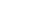,

whereis the depth and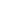is known as the von Kármán
Theodore von Karman
Theodore von Kármán was a Hungarian-American mathematician, aerospace engineer and physicist who was active primarily in the fields of aeronautics and astronautics. He is responsible for many key advances in aerodynamics, notably his work on supersonic and hypersonic airflow characterization...

constant. Thus the gradient can be integrated to solve for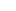: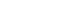.

So we see that the mean flow in the surface layer has a logarithm
Logarithm
The logarithm of a number is the exponent by which another fixed value, the base, has to be raised to produce that number. For example, the logarithm of 1000 to base 10 is 3, because 1000 is 10 to the power 3: More generally, if x = by, then y is the logarithm of x to base b, and is written...

ic relationship with depth.

## The Surface layer in oceanography

The surface layer is studied in oceanography, as both the wind stress
Wind stress
In physical oceanography and fluid dynamics, the wind stress is the shear stress exerted by the wind on the surface of large bodies of water – such as oceans, seas, estuaries and lakes. It is the force component parallel to the surface, per unit area, as applied by the wind on the water surface...

and action of surface waves can cause turbulent mixing necessary for the formation of a surface layer.

The logarithmic flow profile has long been observed in the ocean, but recent, highly sensitive measurements reveal a sublayer within the surface layer in which turbulent eddies are enhanced by the action of surface waves.
It is becoming clear that the surface layer of the ocean is only poorly modeled as being up against the "wall" of the air-sea interaction. Observations of turbulence in Lake Ontario reveal under wave-breaking conditions the traditional theory significantly underestimates the production of turbulent kinetic energy within the surface layer.

### Diurnal cycle

After nighttime convection over the ocean, the turbulent surface layer is found to completely decay and restratify. The decay is caused by the decrease in solar insolation
Insolation
Insolation is a measure of solar radiation energy received on a given surface area in a given time. It is commonly expressed as average irradiance in watts per square meter or kilowatt-hours per square meter per day...

, divergence of turbulent flux and relaxation of lateral gradients.